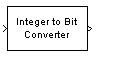# Integer to Bit Converter

Map vector of integers to vector of bits

•Libraries:
Simulink / Logic and Bit Operations
Communications Toolbox / Utility Blocks

## Description

The Integer to Bit Converter block maps each integer (or fixed-point value) in the input vector to a group of bits in the output vector.

This block is single-rate and single-channel. The block maps each integer value (or stored integer when you use a fixed point input) to a group of M bits, using the selection for the Output bit order to determine the most significant bit. The resulting output vector length is M times the input vector length.

## Ports

### Input

expand all

Input signal, specified as an integer or a length N column vector of integers.

If M is specified by the Number of bits per integer(M) parameter:

• When the Number of bits per integer parameter is set to `Unsigned`, input values must be integers in the range [0, (2M – 1)].

• When the Number of bits per integer parameter is set to `Signed`, input values must be integers in the range [(–2M-1), (2M – 1 – 1)].

During simulation, the block performs a run-time check and issues an error if any input value is outside of the appropriate range. When the block generates code, it does not perform this run-time check.

Data Types: `double`

### Output

expand all

Output signal, returned as a scalar or column vector of bits of length M·N.

## Parameters

expand all

Number of input bits mapped to each integer in the input, specified as an integer in the range [1, 32].

#### Programmatic Use

 Block Parameter: `nbits` Type: character vector Values: integer in the range [1, 32] Default: `'3'`

Indicate if the integer value input ranges should be treated as signed or unsigned.

#### Programmatic Use

 Block Parameter: `signedInputValues` Type: character vector Values: `'Unsigned'` | `'Signed'` Default: `'Unsigned'`

Define whether the first bit of the output signal is the most significant bit (MSB) or the least significant bit (LSB).

#### Programmatic Use

 Block Parameter: `bitOrder` Type: character vector Values: `'MSB first'` | `'LSB first'` Default: `'MSB first'`

Specify the data type of the output bits. You can choose one of the following Output data type options:

• `Inherit via internal rule` –– The block determines the output data type based on the input data type.

• If the input signal is floating-point (either `single` or `double`), the output data type is the same as the input data type.

• If the input data type is not floating-point, the output data type is determined as if the parameter is set to `Smallest integer`.

• `Smallest integer` ––The block selects the output data type based on settings used in the Hardware Implementation Pane of the Configuration Parameters dialog box.

• If you select `ASIC/FPGA` for the device vendor, the output data type is the ideal one-bit size (ufix1).

• For all other device vendor selections, the output data type is an unsigned integer with the smallest available word length, as defined in the Hardware Implementation settings (for example, uint8)

• `Same as input`

• `double`

• `single`

• `uint8`

• `uint16`

• `uint32`

#### Programmatic Use

 Block Parameter: `outDtype` Type: character vector Values: ```'Inherit via internal rule'``` | `'Smallest unsigned integer'` | ```'Same as input'``` | `'double'` | `'single'` | `'int8'` | `'uint8'` | `'int16'` | `'uint16'` | `'int32'` | `'uint32'`| `'boolean'` Default: ```'Inherit via internal rule'```

## Block Characteristics

 Data Types `Boolean` | `double` | `fixed pointa` | `integer` | `single` Direct Feedthrough `no` Multidimensional Signals `no` Variable-Size Signals `yes` Zero-Crossing Detection `no` a ufix(1) only at the output when ASIC/FPGA is selected in the Hardware Implementation Pane.

## Version History

Introduced before R2006a

expand all